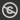Science, Maths & Technology
Free course

# Number theory## Course reviews

This free course is an introduction to Number Theory. Section 1 provides a brief introduction to the kinds of problem that arise in Number Theory. Section 2 reviews and provides a more formal approach to a powerful method of proof, mathematical induction. Section 3 introduces and makes precise the key notion of divisibility. The Division Algorithm, concerning the division of one integer by another, is used. Its consequences, both practical and theoretical, make it a cornerstone of number theory. Section 4 explores some of the basic properties of the prime numbers and introduces the sieve of Eratosthenes.

## Course learning outcomes

After studying this course, you should be able to:

• use, and understand the theoretical underpinnings of, mathematical induction
• understand and be able to apply the Generalised Principle of Mathematical Induction and the Second Principle of Mathematical Induction
• recognise the importance of the Division Algorithm, and be able to apply it in a variety of scenarios
• understand the term 'prime number', and be able to recall basic properties of integers relating to prime numbers
• find all prime numbers in a given range using the sieve of Eratosthenes.

First Published: 09/03/2018

Updated: 04/04/2019

Skip Rate and Review